# Ellipsoidal harmonic

Let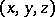be Cartesian coordinates in the Euclidean space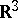, related to the ellipsoidal coordinates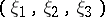by three formulas of the same form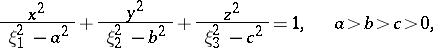where,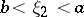and. Putting, one obtains coordinate surfaces in the form of ellipsoids. A harmonic functionthat is a solution of the Laplace equation can be written as a linear combination of expressions of the form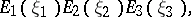(*)
where the factors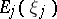,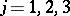, are solutions of the Lamé equation. Expressions of the form (*) for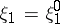and their linear combinations are called ellipsoidal harmonics or, better, surface ellipsoidal harmonics, in contrast to combinations of expressions (*) depending on all three variables, which are sometimes called spatial ellipsoidal harmonics.